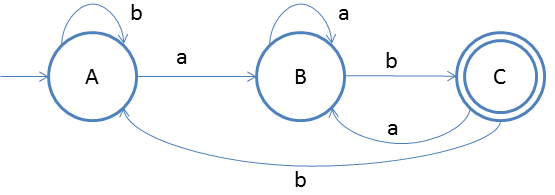# DFA String Examples

Design a DFA in which set of all strings can be accepted which ends with ab.
Given: Input alphabet, Σ={a, b}
Language L ={ab, abab, abaabbab, abbab, bbabaabab ….}

Clearly the language is infinite because there is infinite number of strings.The idea behind this approach is very simple, follow the steps below and you will understand.
1. First we will make DFA for accepting the smallest string that is ‘ab’
2. In DFA we have to take care of all the input alphabets at every state.
3. so we have to take care of input symbol ‘b’ on state A, that is we made self-loop on start state.
4. On state B if a comes then we will accept it as repetition of ‘a’ after a will not ruin anything . Because we want ab in the end.
5. on State C if b comes then that will be a problem as we only want ab in the end not bb, so we will direct b to state A.
6. If ‘a’ comes on state C then we will direct it to state B and if one ‘b’ comes then we will be good by getting ‘ab’ in the end

### Testing

1. Lets take one input string baababab
2. First input is b, so from state A we will go to state A itself
3. Second input is a, so from state A we will go to state B
4. Third input is a, so from state B we will go to state B itself
5. Fourth input is b, so from state B we will go to state C
6. Fifth input is a, so from state C we will go to state B
7. Sixth input is b, so from state B we will go to state C
8. Seventh input is a, so from state C we will go to state B
9. Eighth input is b, so from state B we will go to state C(final state)
After end of the string we are at final state so string is accepted.